# How to convert a datetime.timedelta to String in PythonLast updated: Aug 25, 2023
6 min## #How to convert a datetime.timedelta to String in Python

You can use the `str()` class to convert a `datetime.timedelta` object to a string in Python.

Once you pass the `timedelta` object to the `str()` constructor, its string representation is returned.

main.py
```Copied!```from datetime import datetime

start_datetime = datetime(2023, 9, 20, 8, 30)
end_datetime = datetime(2023, 9, 30, 10, 30)

time_delta = end_datetime - start_datetime

print(time_delta)  # 👉️ 10 days, 2:00:00
print(type(time_delta))  # 👉️ <class 'datetime.timedelta'>

time_delta = str(time_delta)
print(time_delta)  # 👉️ 10 days, 2:00:00
print(type(time_delta))  # 👉️ <class 'str'>
``````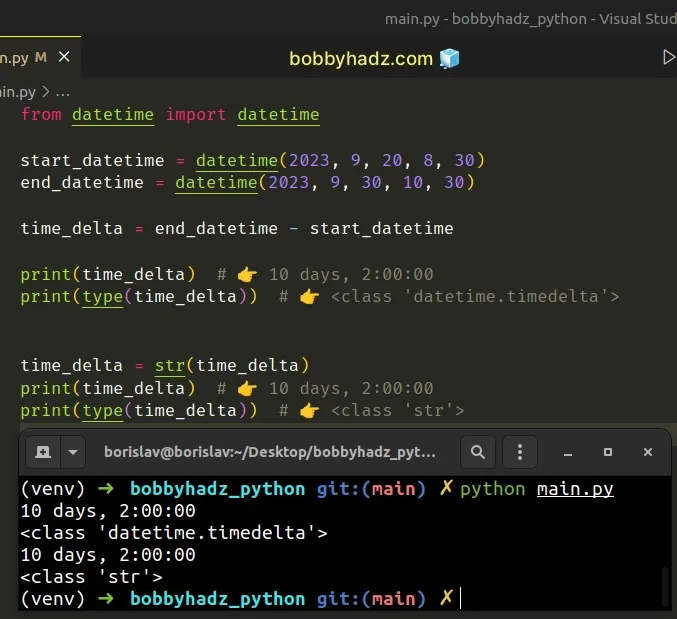1. We subtracted the start `datetime` object from the end `datetime` object to get a `timedelta` object.
2. The str class returns the string representation of the `timedelta` object.
main.py
```Copied!```time_delta = str(time_delta)

print(time_delta)  # 👉️ 10 days, 2:00:00
print(type(time_delta))  # 👉️ <class 'str'>
``````

You can also pass the `datetime.timedelta` object to the print function to print its string representation.

main.py
```Copied!```from datetime import datetime

start_datetime = datetime(2023, 9, 20, 8, 30)
end_datetime = datetime(2023, 9, 30, 10, 30)

time_delta = end_datetime - start_datetime

print(time_delta)  # 👉️ 10 days, 2:00:00
print(type(time_delta))  # 👉️ <class 'datetime.timedelta'>
``````

## #String conversion is necessary if you need to concatenate strings

Converting the `timedelta` object to a string is only necessary if you need to concatenate strings.

main.py
```Copied!```from datetime import datetime

start_datetime = datetime(2023, 9, 20, 8, 30)
end_datetime = datetime(2023, 9, 30, 10, 30)

time_delta = end_datetime - start_datetime

print(time_delta)  # 👉️ 10 days, 2:00:00
print(type(time_delta))  # 👉️ <class 'datetime.timedelta'>

time_delta = str(time_delta)

result = time_delta + ' hours ago'

print(result)  # 👉️ 10 days, 2:00:00 hours ago
``````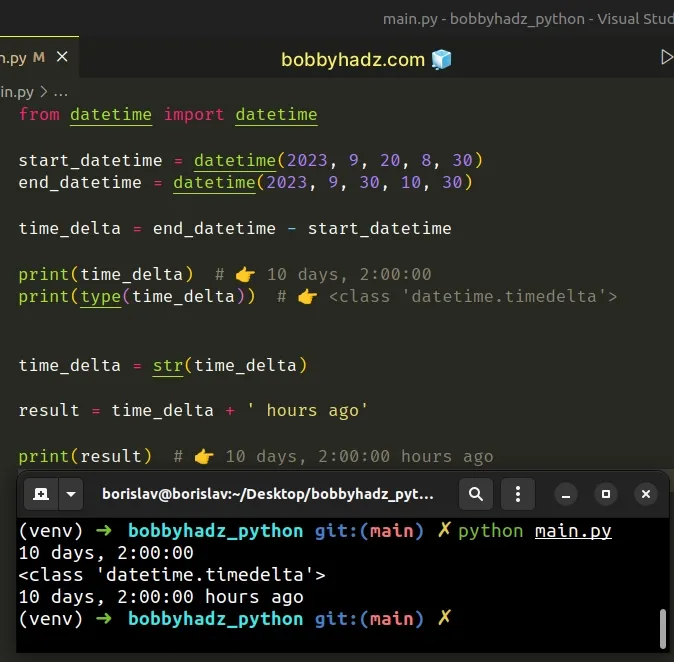The code sample uses the addition (+) operator for concatenation.

## #Using a formatted string literal for implicit string conversion of a `timedelta` object

However, it should be noted that you can also use a formatted string literal to implicitly convert the `timedelta` object to a string when concatenating.

main.py
```Copied!```from datetime import datetime

start_datetime = datetime(2023, 9, 20, 8, 30)
end_datetime = datetime(2023, 9, 30, 10, 30)

time_delta = end_datetime - start_datetime

print(time_delta)  # 👉️ 10 days, 2:00:00
print(type(time_delta))  # 👉️ <class 'datetime.timedelta'>

time_delta = str(time_delta)

result = f'{time_delta} hours ago'

print(result)  # 👉️ 10 days, 2:00:00 hours ago
``````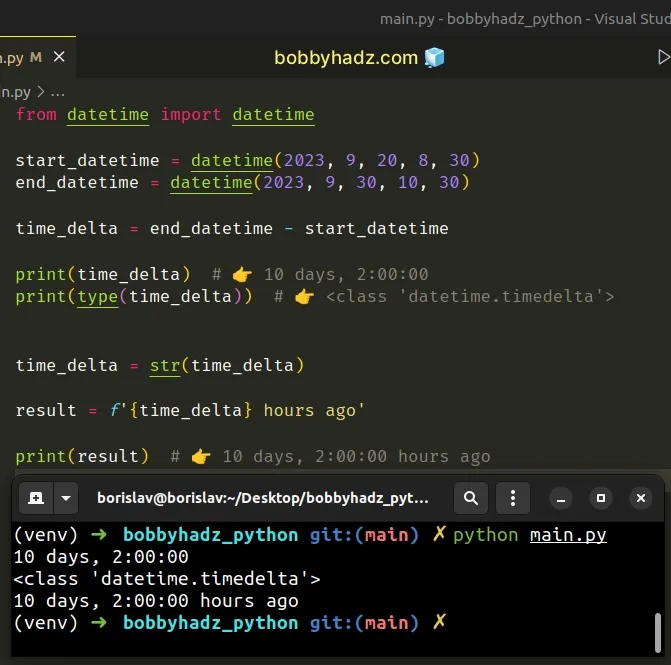Formatted string literals (f-strings) let us include expressions inside of a string by prefixing the string with `f`.

Make sure to wrap expressions in curly braces - `{expression}`.

## #Converting a `timedelta` object to a string to remove the milliseconds

You might also want to convert a `timedelta` object to a string to remove the milliseconds.

main.py
```Copied!```from datetime import datetime

start_datetime = datetime(2023, 9, 20, 8, 30, 15, 453000)
end_datetime = datetime(2023, 9, 30, 10, 30, 15, 421000)

time_delta = end_datetime - start_datetime

print(time_delta)  # 👉️ 10 days, 1:59:59.968000
print(type(time_delta))  # 👉️ <class 'datetime.timedelta'>

time_delta = str(time_delta)
time_delta = time_delta.split('.', maxsplit=1)

print(time_delta)  # 👉️ 10 days, 1:59:59
``````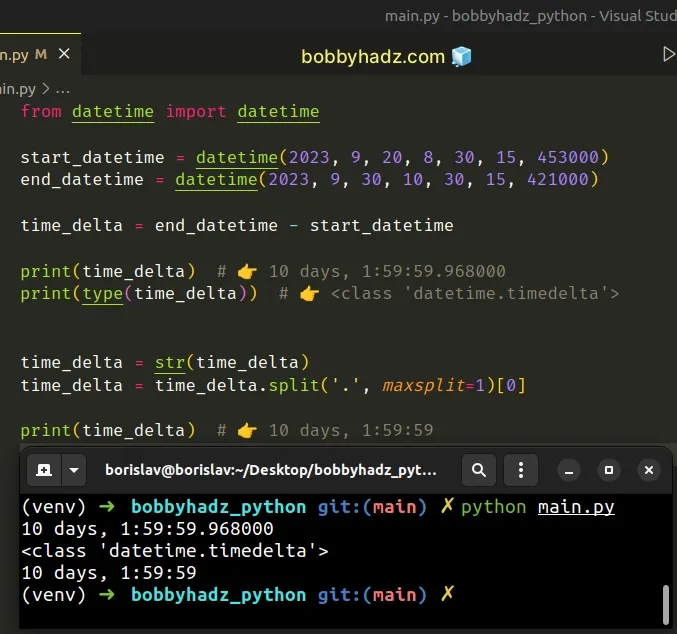We used the `str()` class to convert the `timedelta` object to string to be able to call the str.split() method.

We split the string on the period once and accessed the first list element (index `0`).

Python indexes are zero-based, so the first item in the list has an index of `0` and the last item has an index of `-1` or `len(a_list) - 1`.

## #Formatting the `timedelta` object as a string

If you need to format a `timedelta` object as a string:

1. Use the `seconds` attribute on the `timedelta` to get the total number of seconds.
2. Use the `divmod()` function to calculate the hours, minutes and seconds.
3. Use a formatted string literal to format the hours, minutes and seconds as a string.
main.py
```Copied!```from datetime import datetime

start_datetime = datetime(2023, 9, 20, 8, 30, 40)
end_datetime = datetime(2023, 9, 30, 10, 30, 50)

time_delta = end_datetime - start_datetime

total_seconds = time_delta.seconds
print(total_seconds)  # 👉️ 7200

hours, remainder = divmod(total_seconds, 60 * 60)
minutes, seconds = divmod(remainder, 60)

time_delta_string = f'{hours:02}:{minutes:02}:{seconds:02}'

print(time_delta_string)  # 👉️ 02:00:10
``````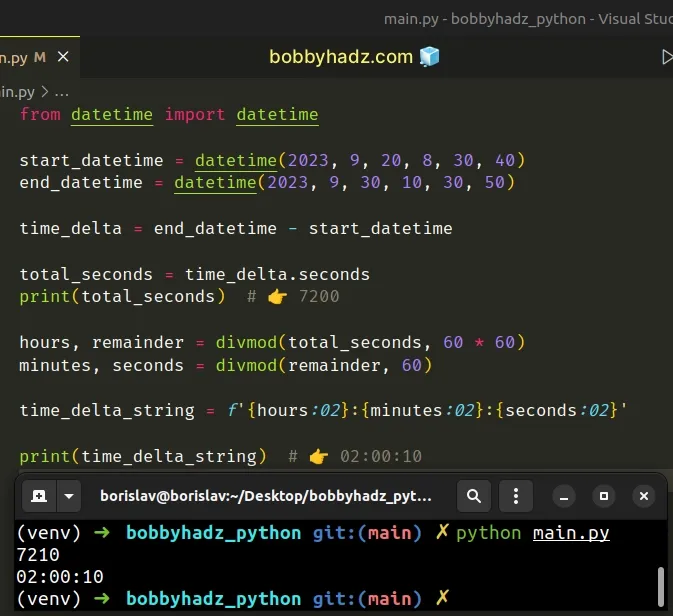The `seconds` attribute on the `timedelta` object stores the total number of seconds it represents.

main.py
```Copied!```total_seconds = time_delta.seconds
print(total_seconds)  # 👉️ 7200
``````

The divmod function takes two numbers and returns a tuple containing 2 values:

1. The result of dividing the first argument by the second.
2. The remainder of dividing the first argument by the second.
main.py
```Copied!```hours, remainder = divmod(total_seconds, 60 * 60)
minutes, seconds = divmod(remainder, 60)
``````

We used the function to calculate the hours, minutes and seconds and used a formatted string literal to format the values.

main.py
```Copied!```time_delta_string = f'{hours:02}:{minutes:02}:{seconds:02}'

print(time_delta_string)  # 👉️ 02:00:10
``````

The hours, minutes and seconds should be displayed as 2 digits, so we added a leading zero if the values are less than 10.

## #Using a reusable function to format the `timedelta` object into a string

You can also use a reusable function to format the `timedelta` object into a string.

main.py
```Copied!```from datetime import datetime

start_datetime = datetime(2023, 9, 20, 8, 30, 40)
end_datetime = datetime(2023, 9, 30, 10, 30, 50)

time_delta = end_datetime - start_datetime
print(time_delta)  # 👉️ 10 days, 2:00:10

def td_format(td_object):
td_components = [
('year', 60 * 60 * 24 * 365),
('month', 60 * 60 * 24 * 30),
('day', 60 * 60 * 24),
('hour', 60 * 60),
('minute', 60),
('second', 1)
]

td_strings = []
total_seconds = int(td_object.total_seconds())

for period, seconds_in_period in td_components:
if total_seconds >= seconds_in_period:
period_value, total_seconds = divmod(
total_seconds,
seconds_in_period
)

ends_with_s = 's' if period_value > 1 else ''

td_strings.append(f'{period_value} {period}{ends_with_s}')

return ', '.join(td_strings)

# 👇️ 10 days, 2 hours, 10 seconds
print(td_format(time_delta))
``````

The function iterates over the list of tuples and:

1. Checks if the total number of seconds in the `timedelta` exceeds the total number of seconds in the period.
2. If the condition is met, the `if` block runs and the period name gets added to the `td_strings` list.
3. If the period value is greater than `1`, then we add an `s` at the end of each period name (e.g. second becomes seconds).
4. The last step is to use the str.join method to join the strings in the list into a single string with a comma separator.

## #Using the `humanize` package to format a `timedelta` in Python

You can also use the humanize package to format a `timedelta` object in Python.

First, open your terminal in your project's root directory and install the package.

shell
```Copied!```pip install humanize

# or with pip3
pip3 install humanize
``````

Now import the module and use it as follows.

main.py
```Copied!```from datetime import datetime
import humanize

start_datetime = datetime(2023, 9, 20, 8, 30, 40, 5000)
end_datetime = datetime(2023, 9, 30, 10, 30, 50, 3000)

time_delta = end_datetime - start_datetime
print(time_delta)  # 👉️ 10 days, 2:00:09.998000

print(humanize.naturaltime(time_delta))  # 👉️ 10 days ago

# 👇️ 10 days, 2 hours and 10.00 seconds
print(humanize.precisedelta(time_delta))

# 👇️ 10 days, 2 hours, 9 seconds and 998 milliseconds
print(
humanize.precisedelta(
time_delta,
minimum_unit="microseconds"
)
)

# 👇️ 242 hours and 9.9980 seconds
print(
humanize.precisedelta(
time_delta,
suppress=["days"],
format="%0.4f"
)
)
``````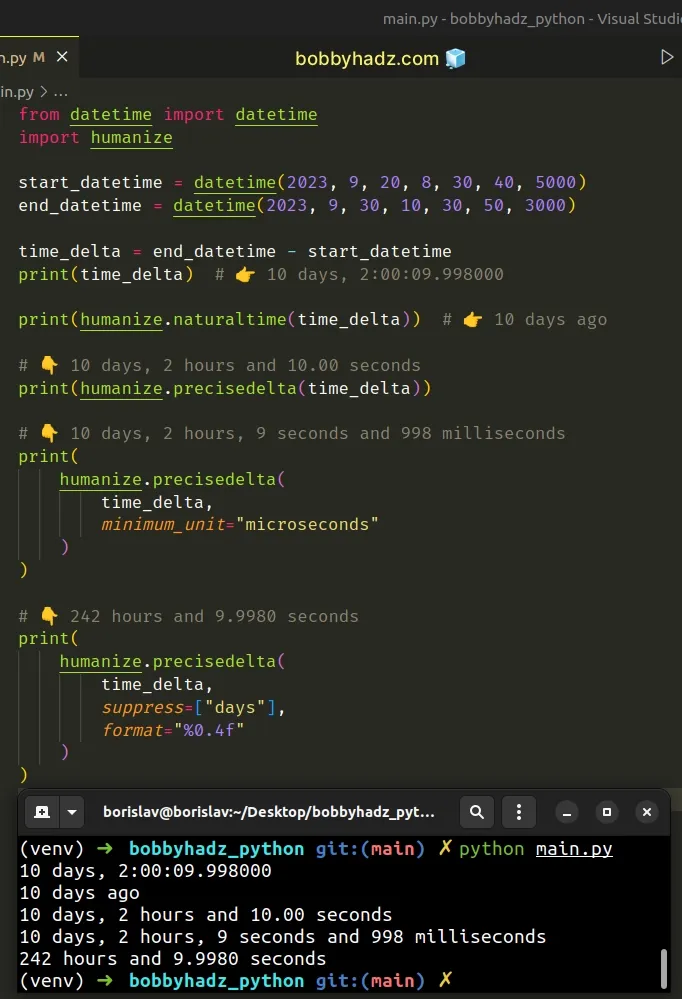The `humanize` package is mostly used to format dates and numeric data into human-readable duration.

## #Getting the hours minutes and seconds by using split

You can also get the hours, minutes and seconds by using `str.split()`.

main.py
```Copied!```from datetime import datetime

start_datetime = datetime(2023, 9, 20, 8, 30, 40)
end_datetime = datetime(2023, 9, 30, 10, 30, 50)

time_delta = end_datetime - start_datetime
print(time_delta)

result = str(time_delta).split(', ', maxsplit=1)
print(result)  # 👉️ 2:00:10
``````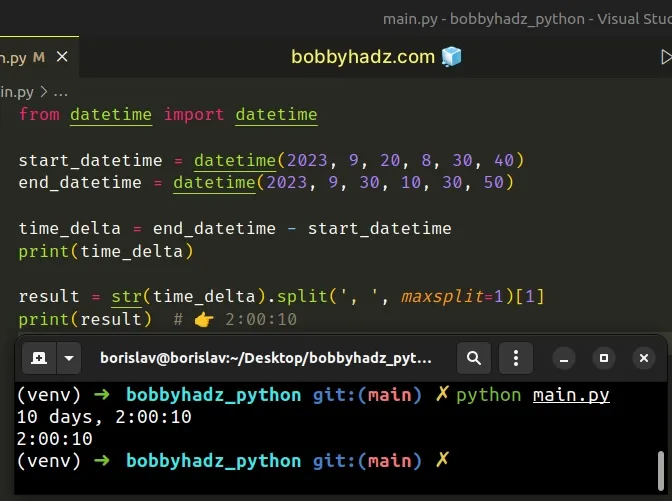1. First, convert the `timedelta` object to a string using the `str()` class.
2. Use the `str.split()` method to split on the comma and space.
3. Access the second list item (index `1`).

Depending on what date/time components your `timedelta` contains the arguments you pass to the `split()` method may differ.